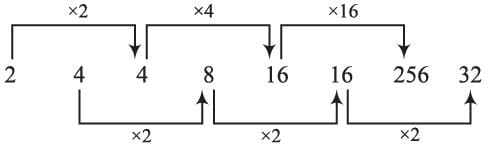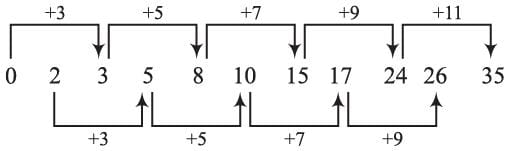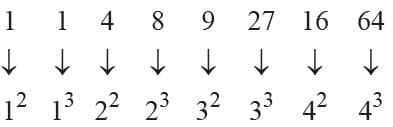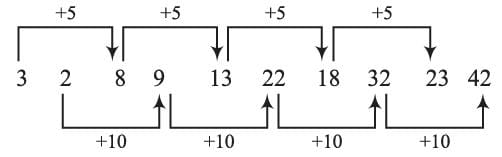Olympiad Test: Series Completion

# Olympiad Test: Series Completion

Test Description

## 15 Questions MCQ Test Science Olympiad Class 9 | Olympiad Test: Series Completion

Olympiad Test: Series Completion for Class 9 2022 is part of Science Olympiad Class 9 preparation. The Olympiad Test: Series Completion questions and answers have been prepared according to the Class 9 exam syllabus.The Olympiad Test: Series Completion MCQs are made for Class 9 2022 Exam. Find important definitions, questions, notes, meanings, examples, exercises, MCQs and online tests for Olympiad Test: Series Completion below.
Solutions of Olympiad Test: Series Completion questions in English are available as part of our Science Olympiad Class 9 for Class 9 & Olympiad Test: Series Completion solutions in Hindi for Science Olympiad Class 9 course. Download more important topics, notes, lectures and mock test series for Class 9 Exam by signing up for free. Attempt Olympiad Test: Series Completion | 15 questions in 15 minutes | Mock test for Class 9 preparation | Free important questions MCQ to study Science Olympiad Class 9 for Class 9 Exam | Download free PDF with solutions
 1 Crore+ students have signed up on EduRev. Have you?
Olympiad Test: Series Completion - Question 1

### There is a series of numbers which follow some definite order. Find the missing term and complete the series. 3, 4, 5, 5, 12, 13, 7, 24, 25, 9, ?, 41

Detailed Solution for Olympiad Test: Series Completion - Question 1

The given numbers are in the set of three numbers which are Pythagorean triplet.
32 + 42 = 52, 52 + 122 = 132,
72 + 242 = 252,  9+ 402 = 412.

Olympiad Test: Series Completion - Question 2

### There is a series of numbers which follow some definite order. Find the missing term and complete the series. 10, 17, 26, 37, 50, …. ?

Detailed Solution for Olympiad Test: Series Completion - Question 2

The pattern of the given series is
32 + 1 = 10;  42 + 1 = 17;  52 + 1 = 26;
62 + 1 = 37;  72 + 1 = 50;
∴ 82 + 1 = 65.

Olympiad Test: Series Completion - Question 3

### There is a series of numbers which follow some definite order. Find the missing term and complete the series. 2, 4, 4, 8, 16, 16, 256, ?

Detailed Solution for Olympiad Test: Series Completion - Question 3∴ ? = 32

Olympiad Test: Series Completion - Question 4

There is a series of numbers which follow some definite order. Find the missing term and complete the series.

1, 5, 7, 14, 18, 20, 40, 44, 46, ?

Detailed Solution for Olympiad Test: Series Completion - Question 4

1 + 4 = 5; 5 + 2 = 7; 7 × 2 = 14
14 + 4  = 18; 18 + 2 = 20; 20 × 2 = 40
40 + 4  = 44; 44 + 2 = 46; 46 × 2 = 92
∴ ? = 92

Olympiad Test: Series Completion - Question 5

There is a series of numbers which follow some definite order. Find the missing term and complete the series.

5, 12, 7, 15, 8, 18, 10, ?

Detailed Solution for Olympiad Test: Series Completion - Question 5

Second, fourth, sixth terms are multiplies of 3 as, 12, 15, 18 and so on the eight-term will be 21.

Olympiad Test: Series Completion - Question 6

There is a series of numbers which follow some definite order. Find the missing term and complete the series.

325, 259, 204, 160, 127, 105, ?

Detailed Solution for Olympiad Test: Series Completion - Question 6

325 – 66 = 259; 259 – 55 = 204;
204 – 44 = 160; 160 – 33 = 127;
127 – 22 = 105; 105 – 11 = 94;
∴ ? = 94

Olympiad Test: Series Completion - Question 7

There is a series of numbers which follow some definite order. Find the missing term and complete the series.

0, 2, 3, 5, 8, 10, 15, 17, 24, 26, ?

Detailed Solution for Olympiad Test: Series Completion - Question 7∴ ? = 35

Olympiad Test: Series Completion - Question 8

There is a series of numbers which follow some definite order. Find the missing term and complete the series.

120, 99, 80, 63, 48, ?

Detailed Solution for Olympiad Test: Series Completion - Question 8

Here
120 – 21 = 99;  99 – 19 = 80; 80 – 17 = 63;
63 – 15 = 48; 48 – 13 = 35;
∴ ? = 35

Olympiad Test: Series Completion - Question 9

There is a series of numbers which follow some definite order. Find the missing term and complete the series.

1, 1, 4, 8, 9, 27, 16, ?

Detailed Solution for Olympiad Test: Series Completion - Question 9∴ ? = 64

Olympiad Test: Series Completion - Question 10

There is a series of numbers which follow some definite order. Find the missing term and complete the series.

10, 14, 26, 42, 70, ?

Detailed Solution for Olympiad Test: Series Completion - Question 10

10 + 14 + 2 = 26; 14 + 26 + 2 = 42;
26 + 42 + 2 = 70; 42 + 70 + 2 = 114;
∴ ? = 114

Olympiad Test: Series Completion - Question 11

In each of the following questions, one term in the number series is wrong. Find out the wrong term.

196, 169, 144, 121, 101.

Detailed Solution for Olympiad Test: Series Completion - Question 11

196 = 142; 169 = 132; 144 = 122;
121 = 112; 102 = 100;
∴ 101 is wrong term.

Olympiad Test: Series Completion - Question 12

In each of the following questions, one term in the number series is wrong. Find out the wrong term.

2, 6, 24, 96, 285, 568, 567

Detailed Solution for Olympiad Test: Series Completion - Question 12

2 × 6 – 6 = 6; 6 × 5 – 5 = 25;
25 × 4 – 4 = 96; 96 × 3 – 3 = 285;
285 × 2 – 2 = 568; 568 × 1 – 1 = 567;
∴ 24 is odd.

Olympiad Test: Series Completion - Question 13

In each of the following questions, one term in the number series is wrong. Find out the wrong term.

93, 309, 434, 498, 521, 533

Detailed Solution for Olympiad Test: Series Completion - Question 13

93 + 63 = 309;  309 + 53 = 434;
434 + 43 = 498;  498 + 32 = 525;
525 + 23 = 533;
∴ 521 is wrong term.

Olympiad Test: Series Completion - Question 14

In each of the following questions, one term in the number series is wrong. Find out the wrong term.

3, 2, 8, 9, 13, 22, 18, 32, 23, 42

Detailed Solution for Olympiad Test: Series Completion - Question 142 + 10 = 12
∴ 9 is the wrong term.

Olympiad Test: Series Completion - Question 15

In each of the following questions, one term in the number series is wrong. Find out the wrong term.

8, 13, 21, 32, 47, 63, 83

Detailed Solution for Olympiad Test: Series Completion - Question 15

8 + 5 = 13; 13 + 8 = 21;
21 + 11 = 32; 32 + 14 = 46;
46 + 17 = 63; 63 + 20 = 83;
∴ 47 is odd.

## Science Olympiad Class 9

17 videos|6 docs|55 tests
 Use Code STAYHOME200 and get INR 200 additional OFF Use Coupon Code
Information about Olympiad Test: Series Completion Page
In this test you can find the Exam questions for Olympiad Test: Series Completion solved & explained in the simplest way possible. Besides giving Questions and answers for Olympiad Test: Series Completion, EduRev gives you an ample number of Online tests for practice

## Science Olympiad Class 9

17 videos|6 docs|55 tests